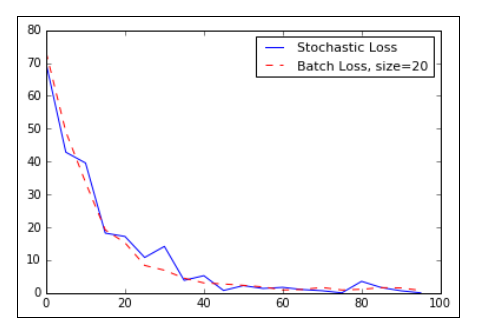# TensorFlow with Batch and Stochastic TrainingTensorFlow with Batch and Stochastic Training. We will show how to extend the prior regression example(TensorFlow Backpropagation), which used stochastic training to batch training.

TensorFlow updates our model variables according to the prior described backpropagation, it can operate on anywhere from one datum observation to a large group of data at once. Operating on one training example can make for a very erratic learning process while using a too large batch can be computationally expensive. Choosing the right type of training is crucial to getting our machine learning algorithms to converge to a solution.

1. In order for TensorFlow to compute the variable gradients for backpropagation to work, we have to measure the loss on a sample or multiple samples.
2. Stochastic training is only putting through one randomly sampled data-target pair at a time.
3. Another option is to put a larger portion of the training examples in at a time and average the loss for the gradient calculation.
4. Batch training size can vary up to and including the whole dataset at once.

We will show how to extend the prior regression example(TensorFlow Backpropagation), which used stochastic training to batch training.

Let’s begin with by loading NumPy , matplotlib , and TensorFlow and start a graph session, as

follows:

``````import matplotlib as plt
import numpy as np
import tensorflow as tf
sess = tf.Session()``````

## What is TensorFlow? TensorFlow

An end-to-end open-source platform for Machine Learning. Before we start with TensorFlow, we will need to know what machine learning and deep learning technologies are.

## A TensorFlow Modeling Pipeline using TensorFlow Datasets and TensorBoard

This article investigates TensorFlow components for building a toolset to make modeling evaluation more efficient. Specifically, TensorFlow Datasets (TFDS) and TensorBoard (TB) can be quite helpful in this task.

## Keras vs. Tensorflow - Difference Between Tensorflow and Keras

Keras vs Tensorflow - Learn the differences between Keras and Tensorflow on basis of Ease to use, Fast development,Functionality,flexibility,Performance etc

## Deployment of a TensorFlow model to Production using TensorFlow Serving

Deploy a Deep Learning Model to Production using TensorFlow Serving.

## A TensorFlow Modeling using TensorFlow Datasets and TensorBoard

While completing a highly informative AICamp online class taught by Tyler Elliot Bettilyon (TEB) called Deep Learning for Developers, I …